# AP Chemistry : Data Analysis and Other Calculations

## Example Questions

2 Next →

### Example Question #11 : Data Analysis And Other Calculations

Working in the laboratory, a student finds the density of pure aluminum to be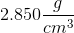. The accepted value for the density of aluminum is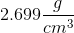. What was the student's percent error?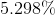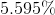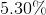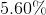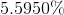Explanation:

To find the percent error, the formula is: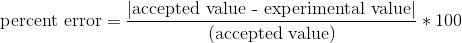Therefore we plug in the values given: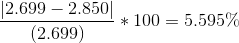Therefore, the correct answer including significant figures is### Example Question #11 : Laboratory Techniques And Analysis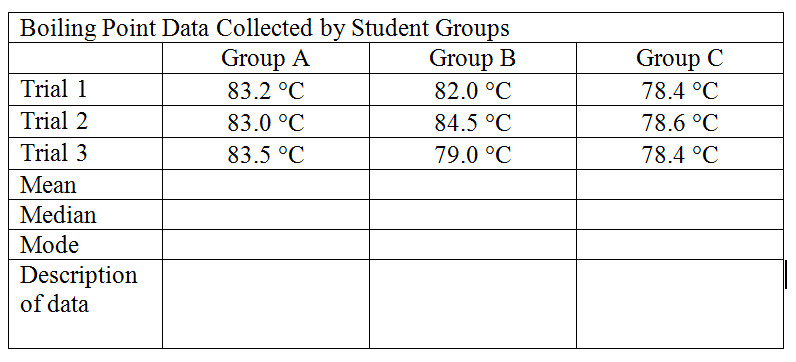The table shows the data obtained by three groups when finding the boiling point of methanol. The accepted value is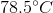. Is group A precise, accurate or both?

Neither precise nor accurate

Precise and accurate

Accurate but not precise

Precise but not accurate

Precise but not accurate

Explanation:

Group A's values are precise because the data values are all close to each other, but it is not accurate because it none of them is near the accepted value.

### Example Question #11 : Laboratory Techniques And AnalysisThe table shows the data obtained by three groups when attempting to find the boiling point of methanol. The accepted value is. Is group C precise, accurate, both, or neither?

Accurate but not precise

Precise and accurate

Neither precise nor accurate

Precise but not accurate

Precise and accurate

Explanation:

Group C is precise because all of their values are close to one another. Their data is also accurate because their values are all near the accepted value. Therefore group C is both accurate and precise.

### Example Question #11 : Laboratory Techniques And Analysis

Find the volume that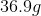of carbon dioxide will occupy if it's density is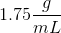.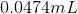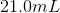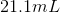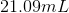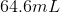Explanation:

Remember: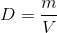Rearrange the equation to solve for volume: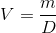Plug in the values given in the problem: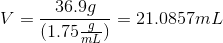However, we must take into account significant figures. Because both our given values have 3 significant figures, our answer must have 3 significant figures.

Therefore after rounding the correct answer is:### Example Question #13 : Laboratory Techniques And Analysis

A student experimentally determines the specific heat of water to be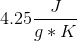. He then looks up the accepted value and finds that it is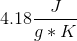. What was the student's percent error?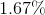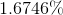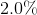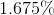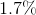Explanation:

The formula for percent error is:Plug in the given values and solve: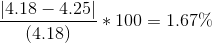Therefore, after accounting for significant figures the correct answer is### Example Question #12 : Laboratory Techniques And Analysis

Place the following metric prefixes in order from smallest to largest:

k, M, m, n

n, m, k, M

m, n, k, M

n, M, k, m

n, k, M, m

n, m, k, M

Explanation:

Remember the saying "King Henry Died by Drinking Chocolate Milk". This is referencing the order of metric prefixes: kilo, hecto, deca, deci, centi, milli. Or in their abbreviations: k, h, da, d, c, m.

You also need to know nano (n) which is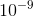.

The final one you need to know for this question is Mega (M) which is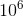Therefore the correct answer is: n, m, k, M (nano, milli, kilo, Mega).

2 Next →

### All AP Chemistry Resources